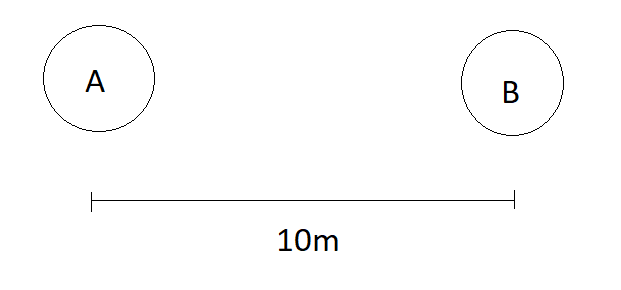# The net gravitational pull!Two objects $A$ and $B$ are separated by a distance of $10m$ (measured from there centers).

If ratio of there masses is $1:4$, then how far should be an object of mass $2kg$ be placed from object $A$ such that net gravitational force on it is $0$.

Note:

• Assume that object $B$ have larger mass than object $A$.

• Submit answer till $2$ digits after decimal point.